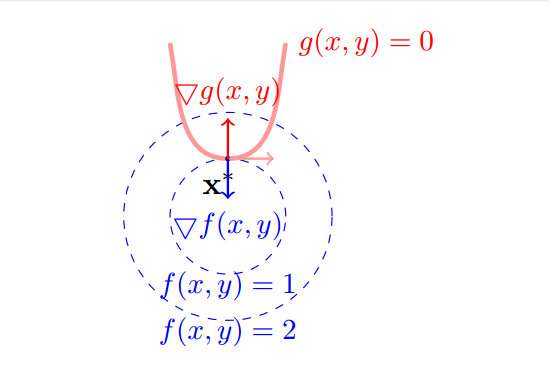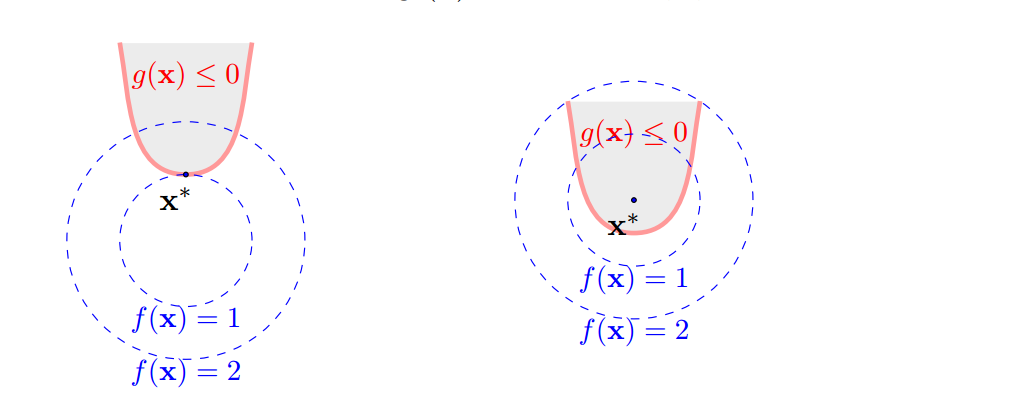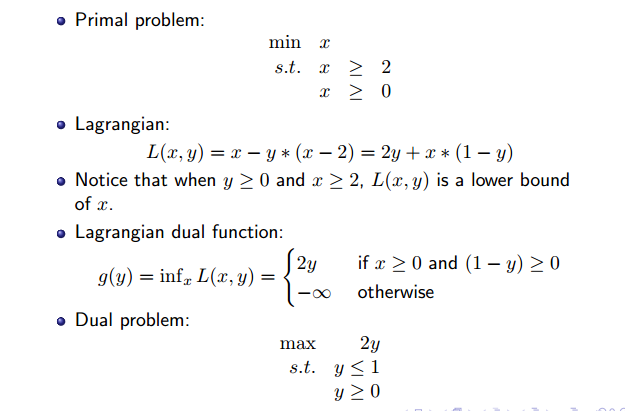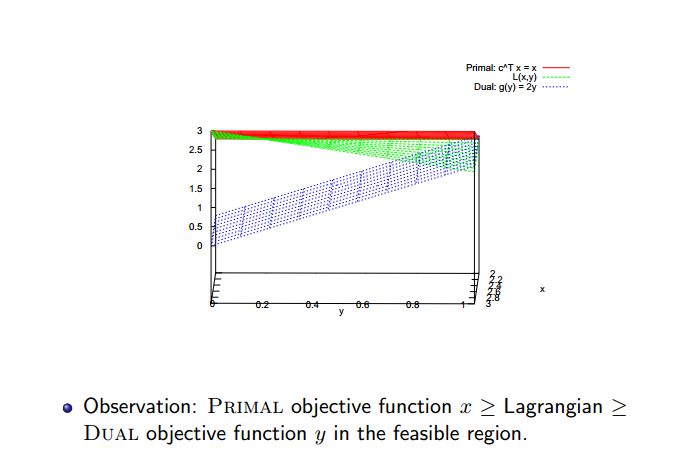# 深度理解拉格朗日乘子法、KKT条件与线性规划对偶理论的微妙关系

## 1.优化问题常见类型

(i) 没有约束条件:

minf(x)

(ii) 有等式约束的优化问题，可以写为:
minf(x)

s.t.gi(x)=0;i=1,...,m

(iii) 再加入不等式约束的优化问题，可以写为

minf(x)

s.t.gi(x)<=0;i=1,...,m

hi(x)=0;i=1,...,p

## 2.拉格朗日乘子法

minf(x,y)

s.t.g(x,y)=0f(x,y)=λg(x,y)

L(x,y,λ)=0

L(x,y,λ)=f(x,y)λg(x,y)

## 3. KKT条件(Karush Kuhn Tucker)

KKT条件其实可以看成是拉格朗日乘子法的进一步推广。对于上面第(iii)类的优化问题，如何求取最优值呢？常用的方法是KKT条件。我们看下面带不等式约束的优化问题:

minf(x)

s.t.gi(x)0,i=1,2,...m1. 如果最优解恰好在这条边界上，那么这个就可以通过拉格朗日来求解。
2. 如果最优解在阴影区内部，我们是要找最优解，这个最优解不在边界上，在他内部，如果在内部那这个解一定是单纯考虑 f(x, y) 的最优解，也就是直接求 f(x,y)=0 $▽f(x, y) = 0$的解。

L(x,λ)=f(x)i=1mλigi(x)

1.(StationaryPoint)L(x,λ)=0 $1.(Stationary Point) ▽L(x^∗, λ) = 0$
2.(PrimalFeasibility)g(x)0 $2. (Primal Feasibility) g(x^∗) ≤ 0$
3.(DualFeasibility)λi0,i=1,2,...,m $3. (Dual Feasibility) λ_i ≤ 0, i = 1,2, ..., m$
4.(ComplementarySlackness)λigi(x)=0,i=1,2,...,m $4. (Complementary Slackness) λ_ig_i(x^∗) = 0, i = 1,2, ..., m$

## 4.从广义拉格朗日(KKT)到线性规划对偶（优化对偶理论）

min:cTx

s.t.Axb

x0

L(x,λ)=cTxi=1mλi(ai1x1+ai2x2+...ainxnbi)

λ>0 $λ>0$时，并且x是可行解的时候， cTxL(x,λ) $c^Tx ≥ L(x, λ)$，即拉格朗日是原问题的一个下界。再进一步讨论我们有下面不等式成立：
cTxL(x,λ)infxL(x,λ)(1)

infxL(x,λ)=infx(cTxmi=1λi(ai1x1+ai2x2+...ainxnbi)) $inf_{x}L(x, λ)=inf_{x} { (c^Tx − \sum_{i=1}^m λ_i(a_{i1}x_1 + a_{i2}x_2 + ...a_{in}x_n − b_i))}$

=infx[λTb+(cTλTA)x] $=inf_{x}[λ^Tb + (c^T − λ^TA)x]$

={λTb,,if cTλTA and x0otherwise $= \begin{cases} λ^Tb, & \text{if c^T ≥ λ^TA and x≥0} \\ -\infty, & \text{otherwise} \end{cases}$

g(λ)=infxL(x,λ)

cTxL(x,λ)g(λ)

g(λ)=λTb $g(λ)=λ^Tb$是当 cTλTA $c^T ≥ λ^TA$ and x0 $x≥0$情况下，函数 f(x) $f(x)$的下边界。也就是说：如果我们要求 λ0 $λ ≥ 0$，并且 x $x$ 是可行解的话，那么我们原先的目标函数肯定比L(x,λ)$L(x, λ)$要大。而 L(x,λ) $L(x, λ)$一定大于等于 g(λ) $g(λ)$。这里的 g(λ) $g(λ)$ 就是拉格朗日对偶。

max:λTb

s.t.λTAcT

λ0

## 4.线性规划原问题与对偶问题(1).对偶的对偶就是原问题
(2).对于一般问题，对偶问题的任意一个可行解总是原问题的一个下界,也叫弱对偶性
(3).对于线性规划，原问题的存在最优解，那对偶问题也存在一个最优解，它们的数值相等，也叫强对偶性
(4).互补松弛性:假如 x 是原问题的可行解， y 为对偶问题的可行解，那么 x 和 y 是最优解时，当且仅当：
1imui=yi(ai1x1+ai2x2+...+ainxnbi)=0 $1 ≤ i ≤ m， u_i = y_i(a_{i1}x_1 + a_{i2}x_2 + ... + a_{in}x_n − b_i) = 0$
1jnvj=(cja1jy1a2jy2...amjym)xj=0 $1 ≤ j ≤ n， v_j = (c_j − a_{1j}y_1 − a_{2j}y_2 − ... − a_{mj}y_m)x_j = 0$01-27
08-16
05-295万+
03-282万+
10-249204
04-062万+
07-3152
02-05369
10-242万+
08-108219
08-011万+
06-271221
01-143623
08-12473
06-0216万+
07-073273
12-035374
©️2020 CSDN 皮肤主题: 编程工作室 设计师:CSDN官方博客